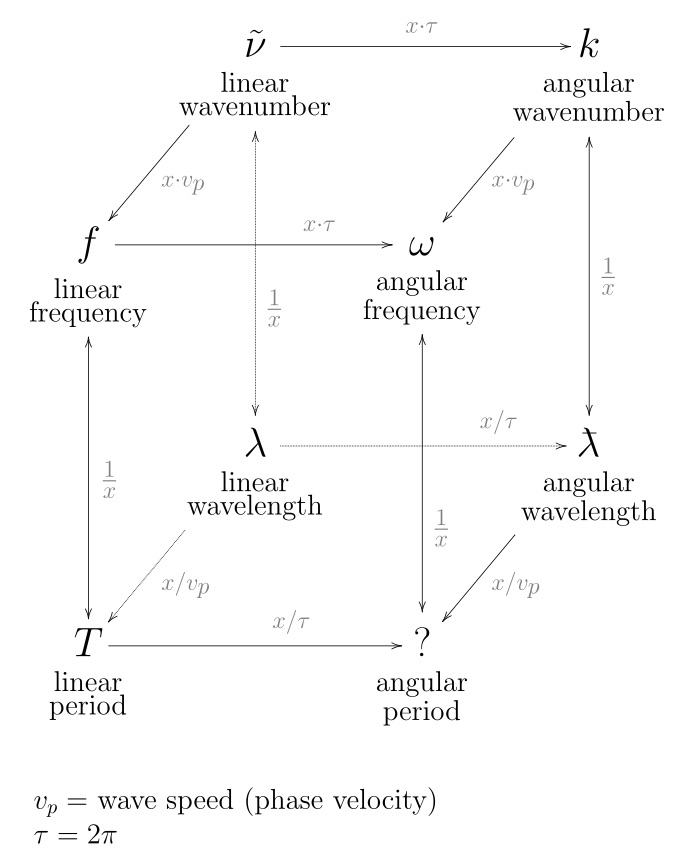# frequencyFrequency is the number of occurrences of a repeating event per unit of time. It is also referred to as temporal frequency, which emphasizes the contrast to spatial frequency and angular frequency. The period is the duration of time of one cycle in a repeating event, so the period is the reciprocal of the frequency. For example, if a newborn baby's heart beats at a frequency of 120 times a minute, its period — the time interval between beats — is half a second (that is, 60 seconds divided by 120 beats). Frequency is an important parameter used in science and engineering to specify the rate of oscillatory and vibratory phenomena, such as mechanical vibrations, audio signals (sound), radio waves, and light. Wikipedia, Frequency

Frequency is the number of cycles per TIME.
Wave Number is the number of cycles per SPACE.
Wavelength is the distance between consecutive corresponding points of the same phase.

Ramsay
It is a remarkable fact that the numbers for the lengths of strings producing the major scale are the number of the vibration of the notes of the minor scale; for example, string-length as 26 2/3 will give the vibrations for [Scientific Basis and Build of Music, page 87]

Nikola Tesla Frequency alone in reality does not mean anything, except when an undisturbed harmonic oscillation is considered. - [Nikola Tesla]

• Number of cycles per unit time. SI unit: hertz, Hz (cycles/s). [Applied Spectroscopy, Vol. 29, #1, 1975]
• The number of vibrations per second; expressed in hertz (Hz). [The Science of Sound]
• Rate at which a waveform repeats. Expressed in cycles per second or Hertz (Hz). [Learning Music with Synthesizers]
• The number of cyclical variations per unit time. Frequency is generally expressed in Hertz (Hz) also denoted cycles per second (cps). [Compendium of Noise Engineering - Part I]

"The repetition rate of a periodic vibration within a unit time. This is normally expressed in units of cycles per minute (cpm), events per minute (epm), or cycles per second (cps or Hz). It can be expressed relative to shaft rotative speed. With respect to rotating machinery vibrations, there are two types of frequencies of interest: A: the shaft rotative frequency, and B: the various vibration frequencies as measured by vibration transducers. Rotative frequency is usually expressed in terms of rpm. Vibration frequencies are commonly expressed in terms of a fraction or percentage of the shaft rotative frequency: 1X means one times rotative frequency, 2X means two times, 1/2X, or 50 percent, means one-half times, etc." [Field of Rotating Machinery Measurement, Monitoring and Analysis]

Christ Returns - Speaks His Truth
"Visible things are but a manifestation of specific frequencies of vibration in consciousness which produces a 'SHIMMER OF MOTES OR PARTICLES' giving an appearance of solid 'matter'.
Each visible substance possesses its own unique vibrational frequency. A change in the rate of vibration produces a change in the appearance of 'matter'. As consciousness energies change so do the appearances of 'matter' change." [Christ Returns - Speaks His Truth, Letter 3, page 19] [Christ Returns - Speaks His Truth, Letter 3, page 19]